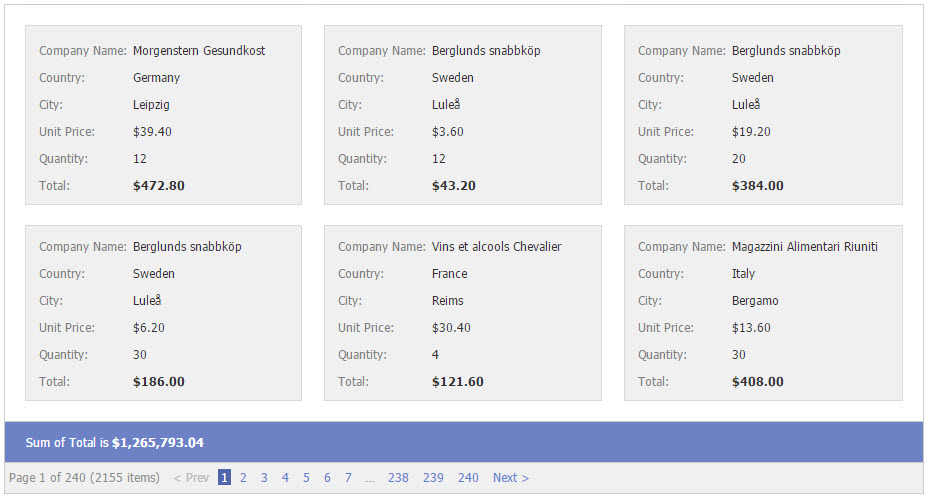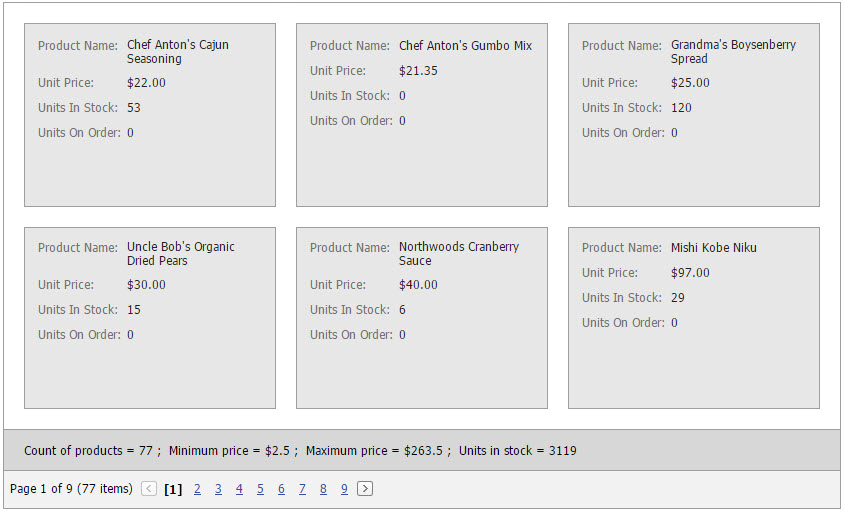# Data Summaries (Card View)

ASPxCardView allows you to display a summary of data columns. For example, in a data summary, you can display the total number of records or the maximum value of a record set.

ASPxCardView supports the total summary featurep, which displays the value of an aggregate function - calculated across all cards within the card view - in the Summary Panel.Summaries are represented by ASPxSummaryItem objects. To work properly, the following two summary item properties must be specified.

``````<dx:ASPxSummaryItem FieldName="Total" SummaryType="Sum"/>
``````

The summary item above summarizes the ‘Total’ field values within cards and displays the sum in the summary panel.

Note

In server mode, a summary cannot be calculated for unbound columns in which values are calculated using events (see ASPxCardView.CustomUnboundColumnData). Only columns with unbound expressions (see CardViewColumn.UnboundExpression) support summary calculation.

## Example

The code sample below demonstrates how to specify total summaries.

The image below shows the result.``````<dx:ASPxCardView ID="ASPxCardView1"  runat="server" AutoGenerateColumns="False" DataSourceID="SqlDataSource1" KeyFieldName="ProductID">
<Settings ShowSummaryPanel="True" />
<Columns>
...
</Columns>
<TotalSummary>
<dx:ASPxCardViewSummaryItem FieldName="ProductID" SummaryType="Count" DisplayFormat="Count of products = {0} " />
<dx:ASPxCardViewSummaryItem FieldName="UnitPrice" SummaryType="Min" DisplayFormat="Minimum price = \${0} " />
<dx:ASPxCardViewSummaryItem FieldName="UnitPrice" SummaryType="Max" DisplayFormat="Maximum price = \${0} " />
<dx:ASPxCardViewSummaryItem FieldName="UnitsInStock" SummaryType="Sum" DisplayFormat="Units in stock = {0} " />
</TotalSummary>
</dx:ASPxCardView>
``````